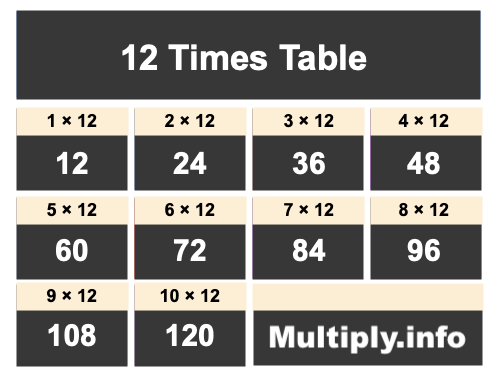12 Times TableThe picture above shows the first ten numbers in the 12 Times Table. It is created my multiplying 12 by one, then by two, then by three, and so on all the way up to ten.

Students and others use our 12 Times Table for many things, such as to find patterns or for memorizing products of numbers multiplied by 12.

In addition to the image of the 12 Times Table above, we have also written the 12 Times Table below showing 12 multiplied by every number up to one hundred. The list is in ascending order.

We created the 12 Times Table below to make it easy to read and print. We hope you will find our 12 Times Table helpful.

12 x  0 = 0
12 x  1 = 12
12 x  2 = 24
12 x  3 = 36
12 x  4 = 48
12 x  5 = 60
12 x  6 = 72
12 x  7 = 84
12 x  8 = 96
12 x  9 = 108
12 x 10 = 120
12 x 11 = 132
12 x 12 = 144
12 x 13 = 156
12 x 14 = 168
12 x 15 = 180
12 x 16 = 192
12 x 17 = 204
12 x 18 = 216
12 x 19 = 228
12 x 20 = 240
12 x 21 = 252
12 x 22 = 264
12 x 23 = 276
12 x 24 = 288
12 x 25 = 300
12 x 26 = 312
12 x 27 = 324
12 x 28 = 336
12 x 29 = 348
12 x 30 = 360
12 x 31 = 372
12 x 32 = 384
12 x 33 = 396
12 x 34 = 408
12 x 35 = 420
12 x 36 = 432
12 x 37 = 444
12 x 38 = 456
12 x 39 = 468
12 x 40 = 480
12 x 41 = 492
12 x 42 = 504
12 x 43 = 516
12 x 44 = 528
12 x 45 = 540
12 x 46 = 552
12 x 47 = 564
12 x 48 = 576
12 x 49 = 588
12 x 50 = 600
12 x 51 = 612
12 x 52 = 624
12 x 53 = 636
12 x 54 = 648
12 x 55 = 660
12 x 56 = 672
12 x 57 = 684
12 x 58 = 696
12 x 59 = 708
12 x 60 = 720
12 x 61 = 732
12 x 62 = 744
12 x 63 = 756
12 x 64 = 768
12 x 65 = 780
12 x 66 = 792
12 x 67 = 804
12 x 68 = 816
12 x 69 = 828
12 x 70 = 840
12 x 71 = 852
12 x 72 = 864
12 x 73 = 876
12 x 74 = 888
12 x 75 = 900
12 x 76 = 912
12 x 77 = 924
12 x 78 = 936
12 x 79 = 948
12 x 80 = 960
12 x 81 = 972
12 x 82 = 984
12 x 83 = 996
12 x 84 = 1008
12 x 85 = 1020
12 x 86 = 1032
12 x 87 = 1044
12 x 88 = 1056
12 x 89 = 1068
12 x 90 = 1080
12 x 91 = 1092
12 x 92 = 1104
12 x 93 = 1116
12 x 94 = 1128
12 x 95 = 1140
12 x 96 = 1152
12 x 97 = 1164
12 x 98 = 1176
12 x 99 = 1188
12 x 100 = 1200

Times Table Charts
Enter another number below to see the Times Table for that number.

13 Times Table
Did you find useful patterns in our 12 Times Table that you can use for the future? Check out the next Times Table on our list.